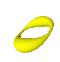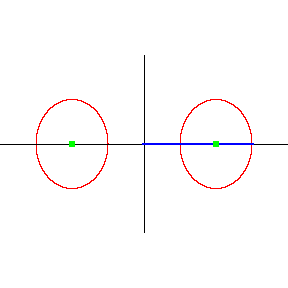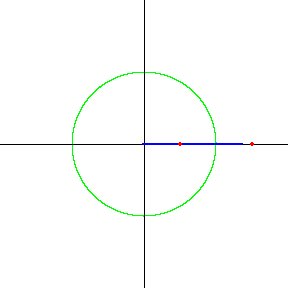Summer 2004

# Mathematics Maths21a Summer 2004

## Multivariable Calculus

Office: SciCtr 434
Email: knill@math.harvard.edu## Torus Parametrization Hint (Problem 5 from the Lesson 6 HW)

 Here is a hint to Problem 5 in the 6.th lesson Problem set: We keep the angle theta as one of the parameters and let r the distance of a point on the torus to the z-axis. This distance is r=2+cos(phi) if phi is the angle you see on the animated figure below to the left. Note that phi has no relations with the angle phi in spherical coordinates. The blue segment you see has the length r. You can read off from the same (left) picture also that z=sin(phi). To finish the parametrization problem, you have to translate back from cylindrical coordinates (r,theta,z)=(2+cos(phi),theta,sin(phi)) to Cartesian coordinates (x,y,z). Write down your result in the form r(theta,phi)= (x(theta,phi),y(theta,phi), z(theta,phi)).Changing the angle phi. In this picture the vertical axes is the z-axes. This picture obtained by cutting through the doughnut. For example along the xz-plane. Changing the angle theta. In this picture the axes are the x and y axes. You look onto the doughnut from above.